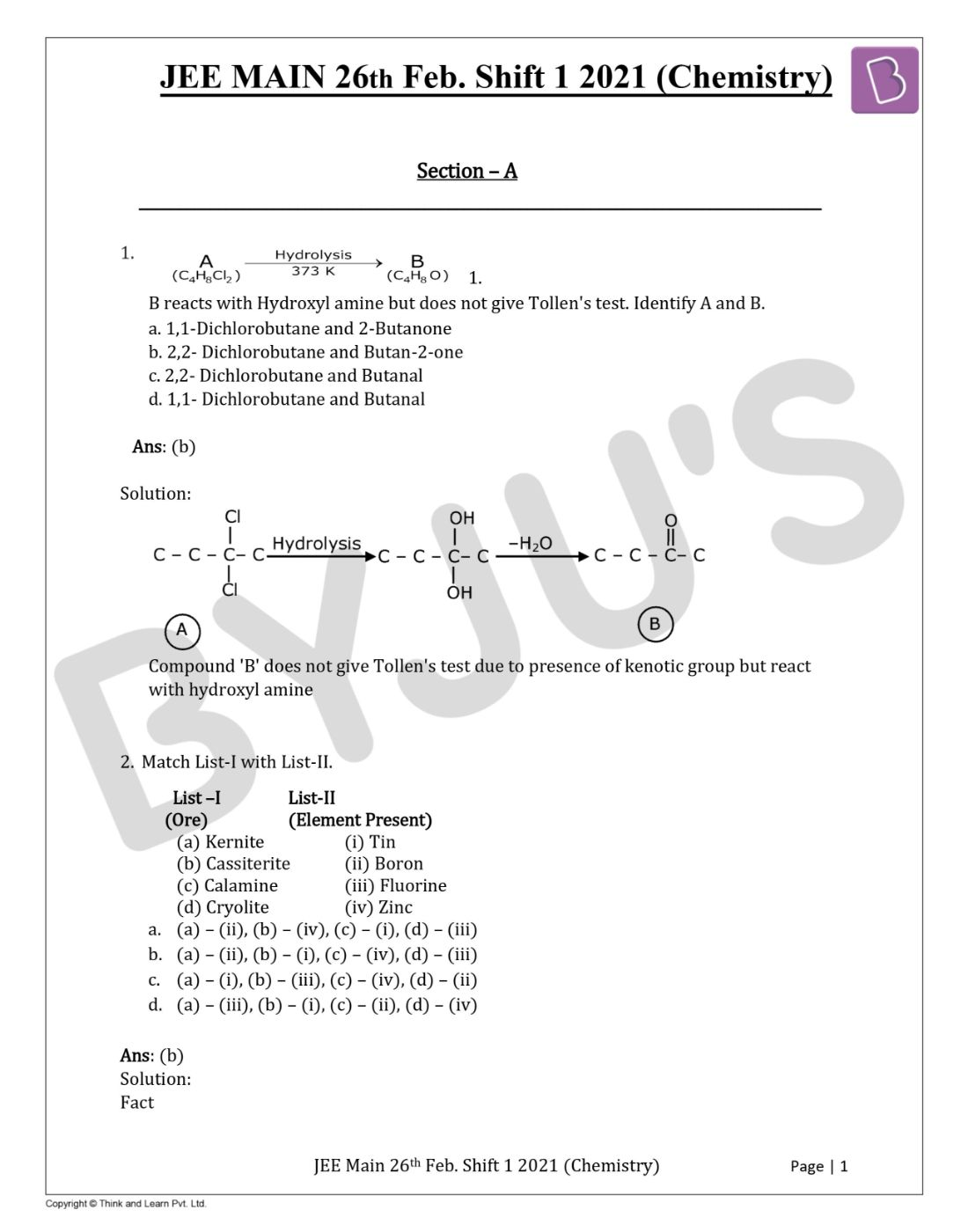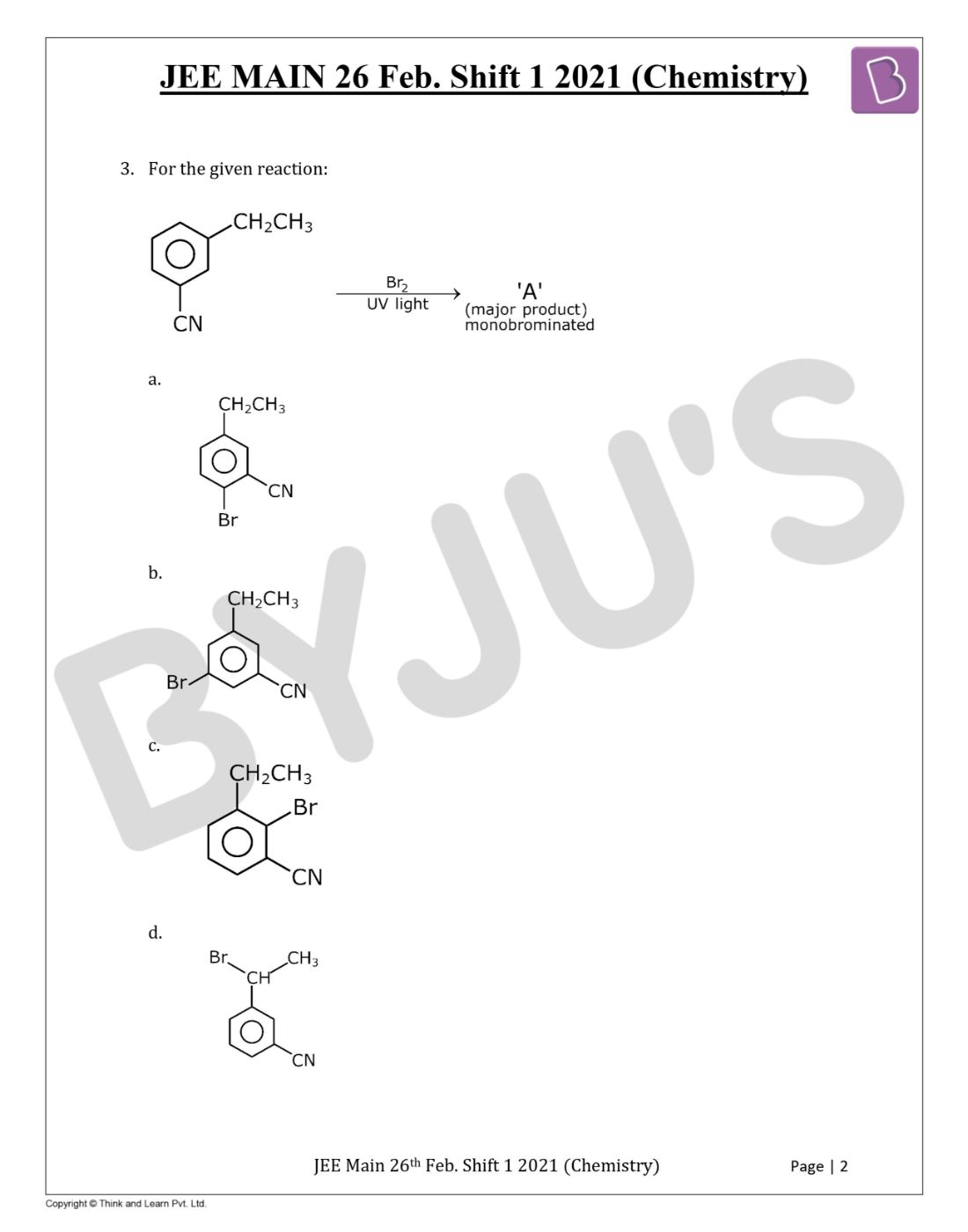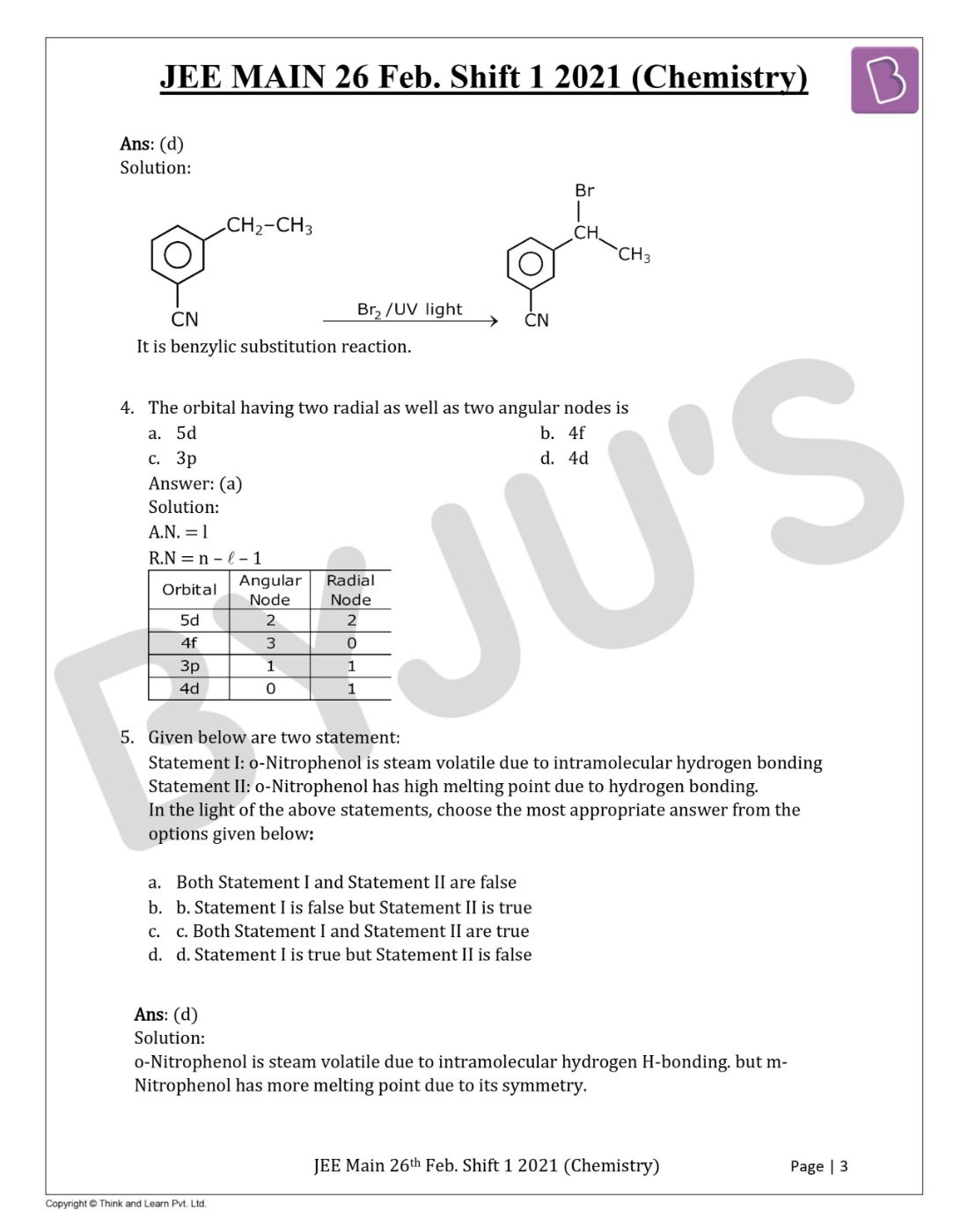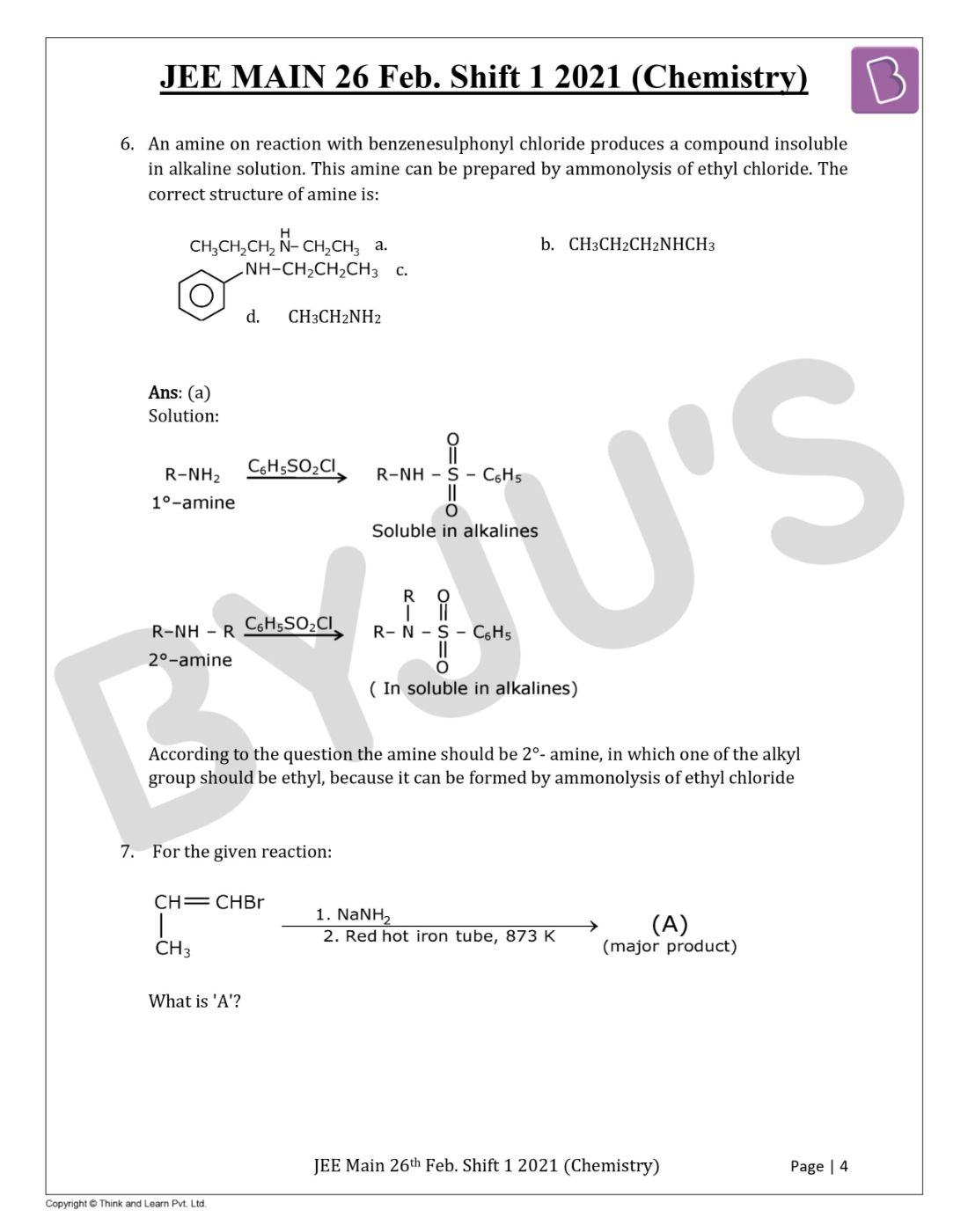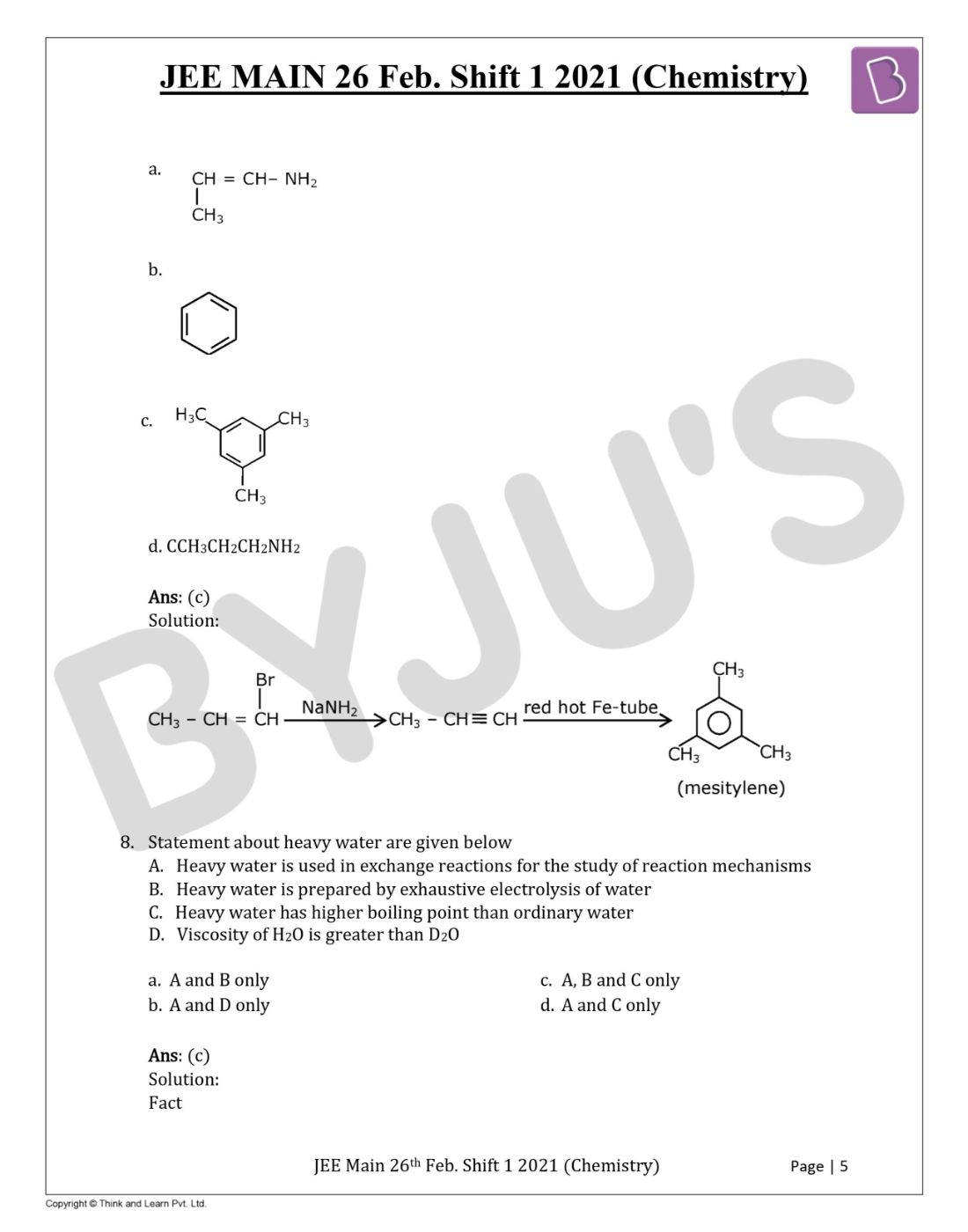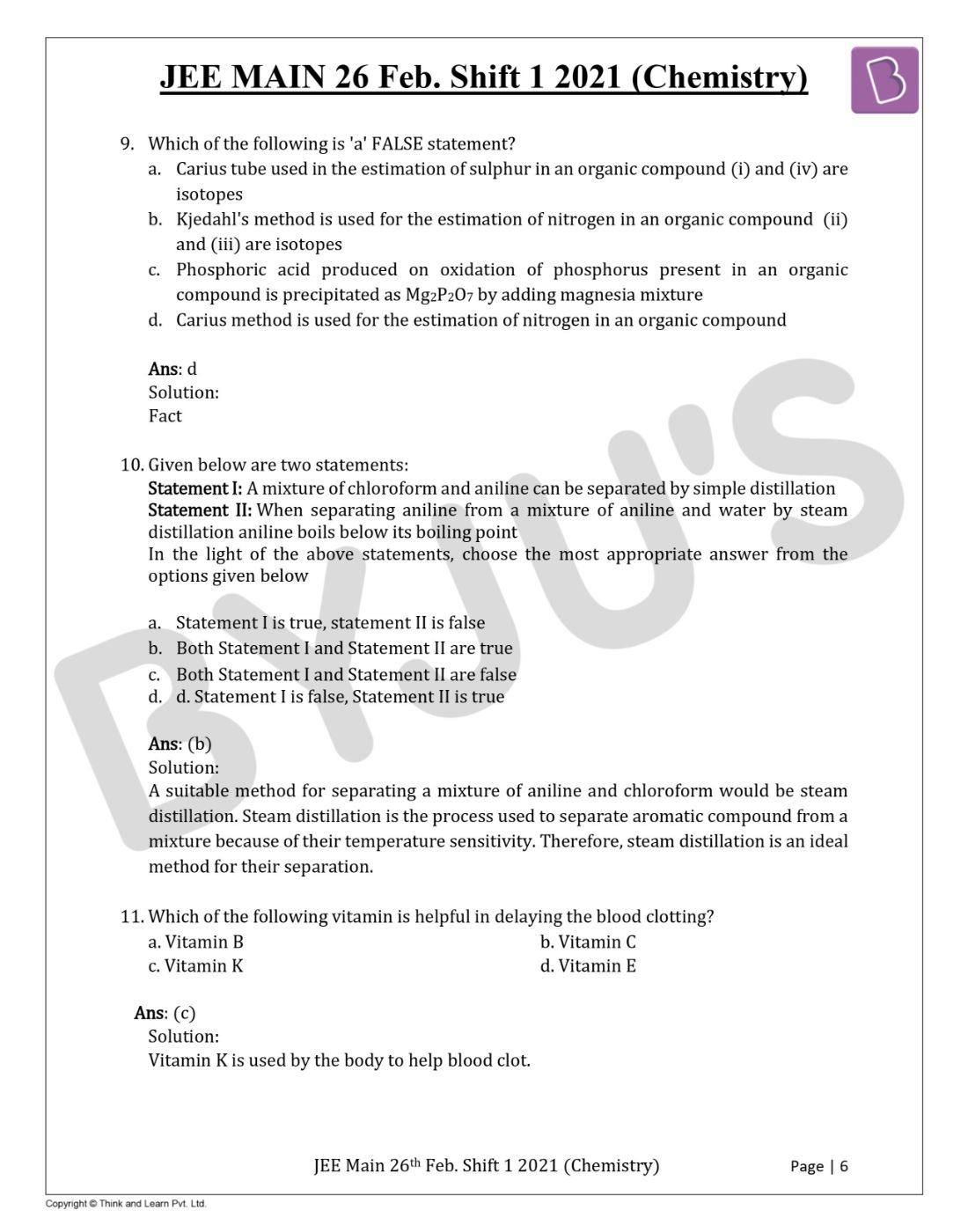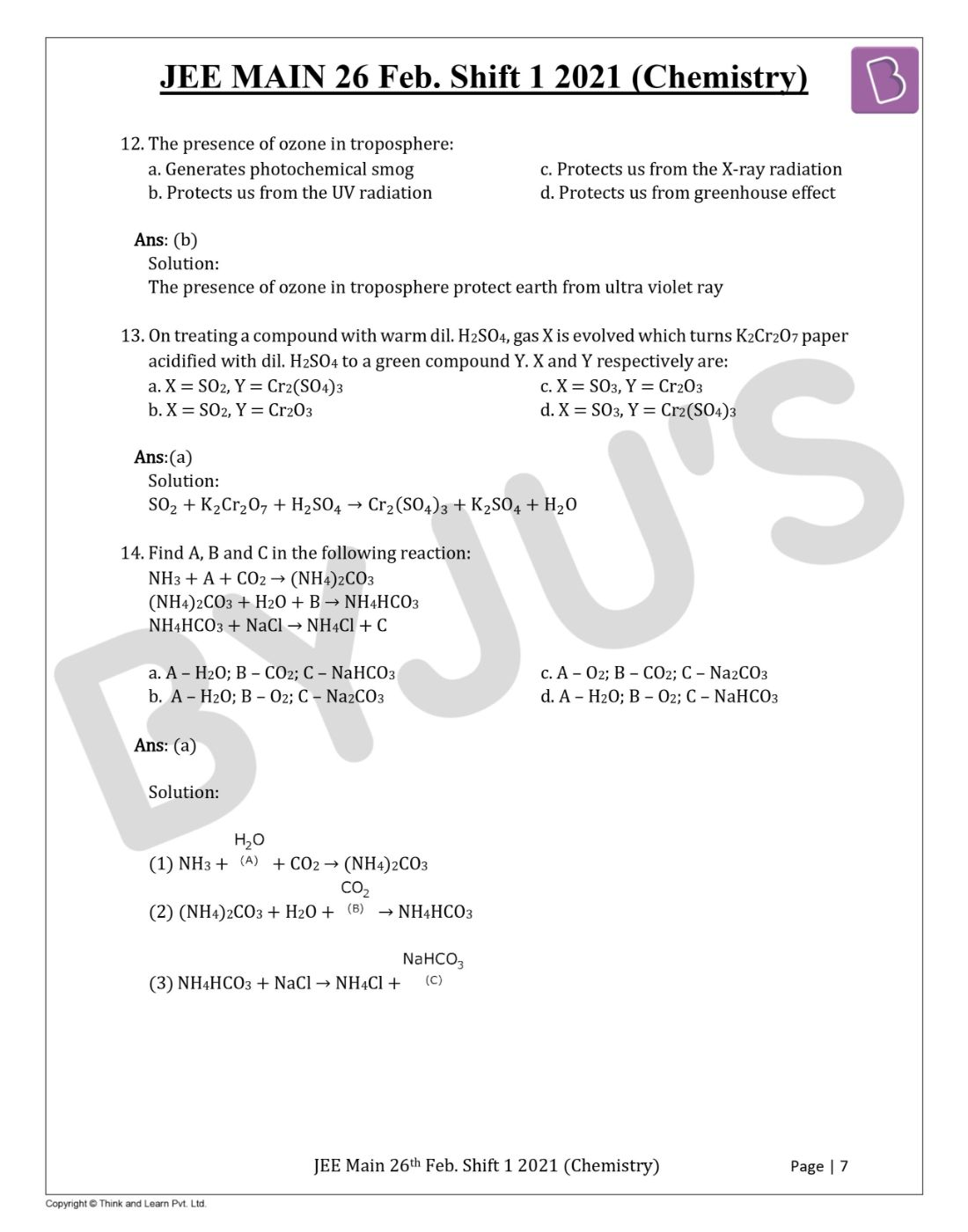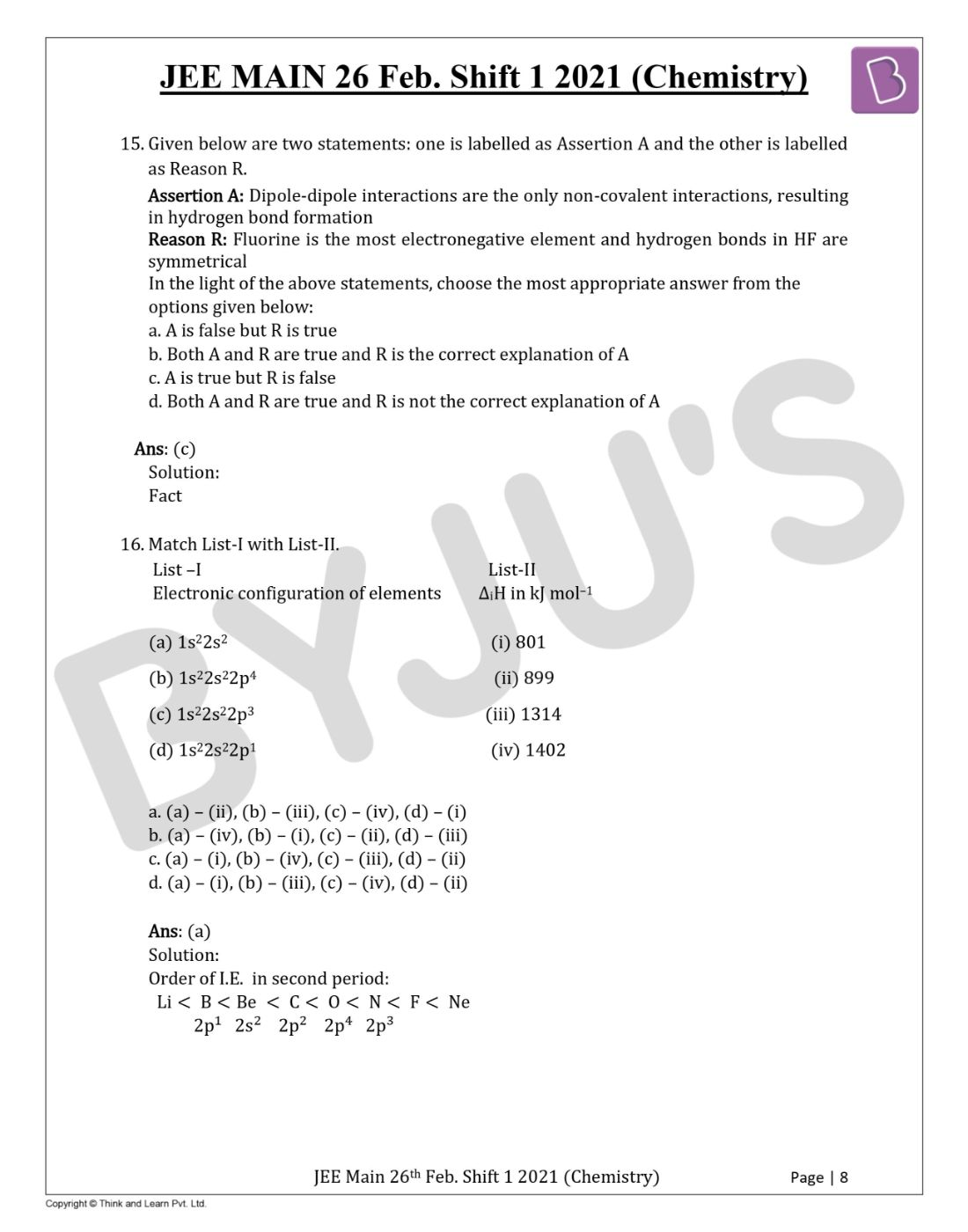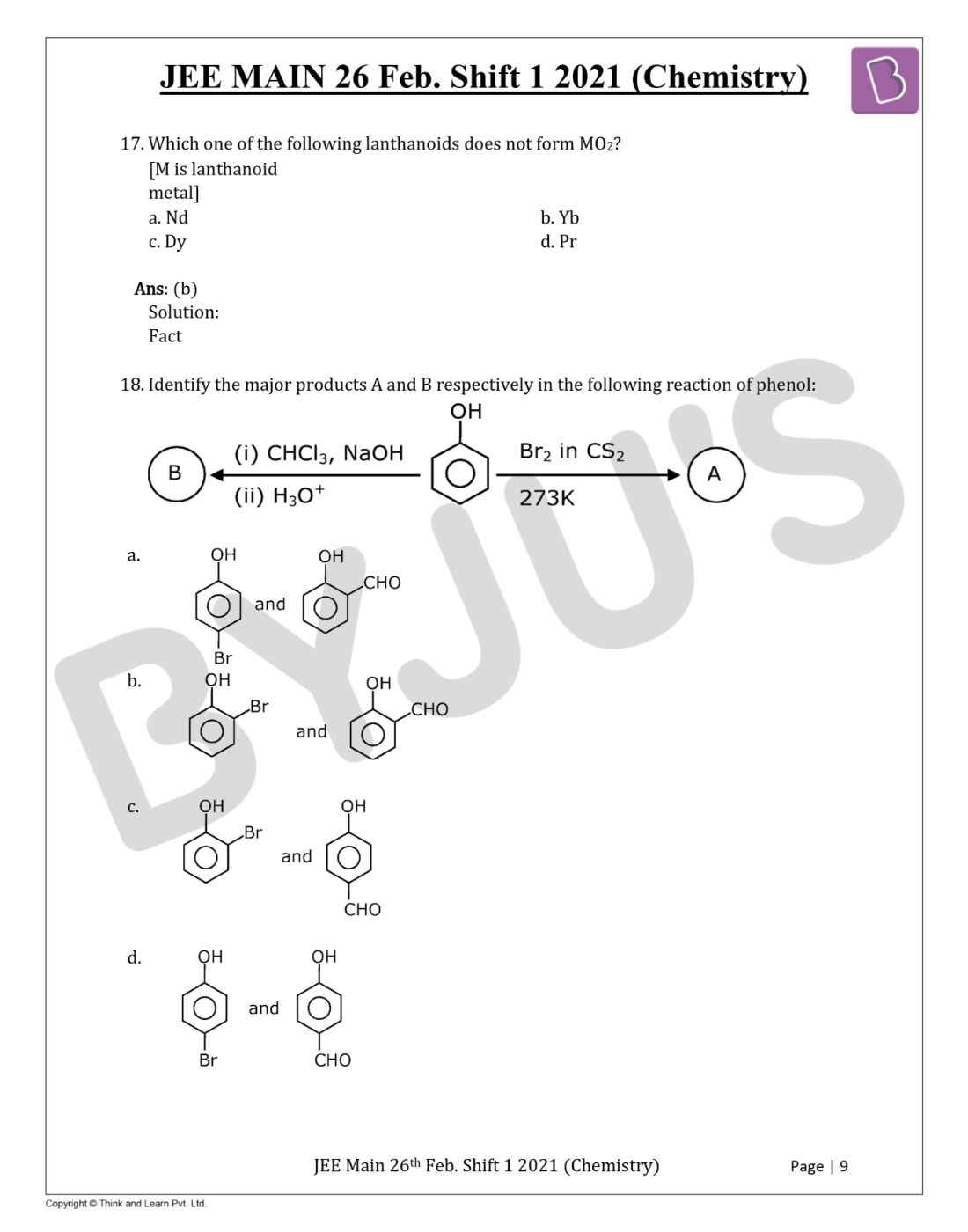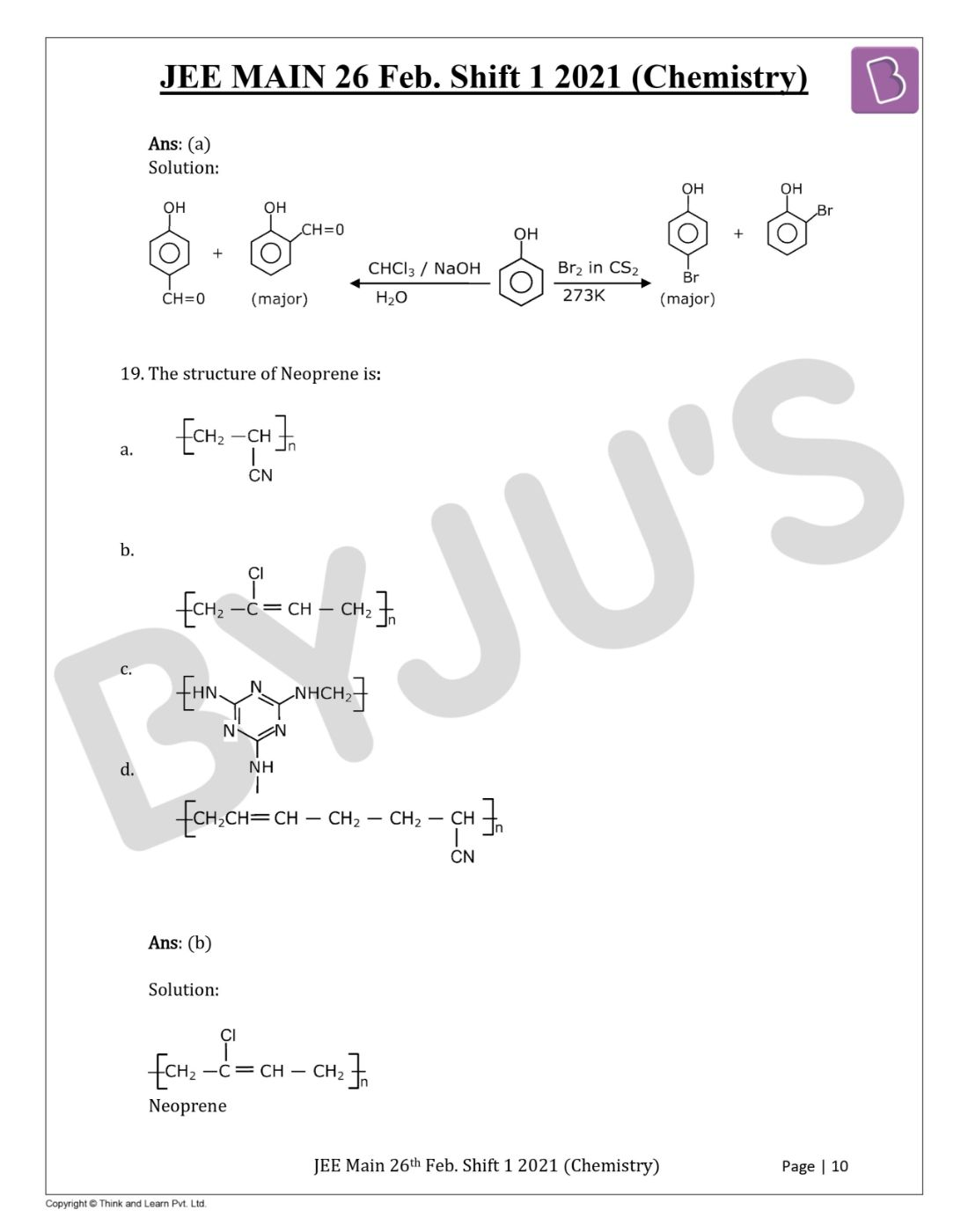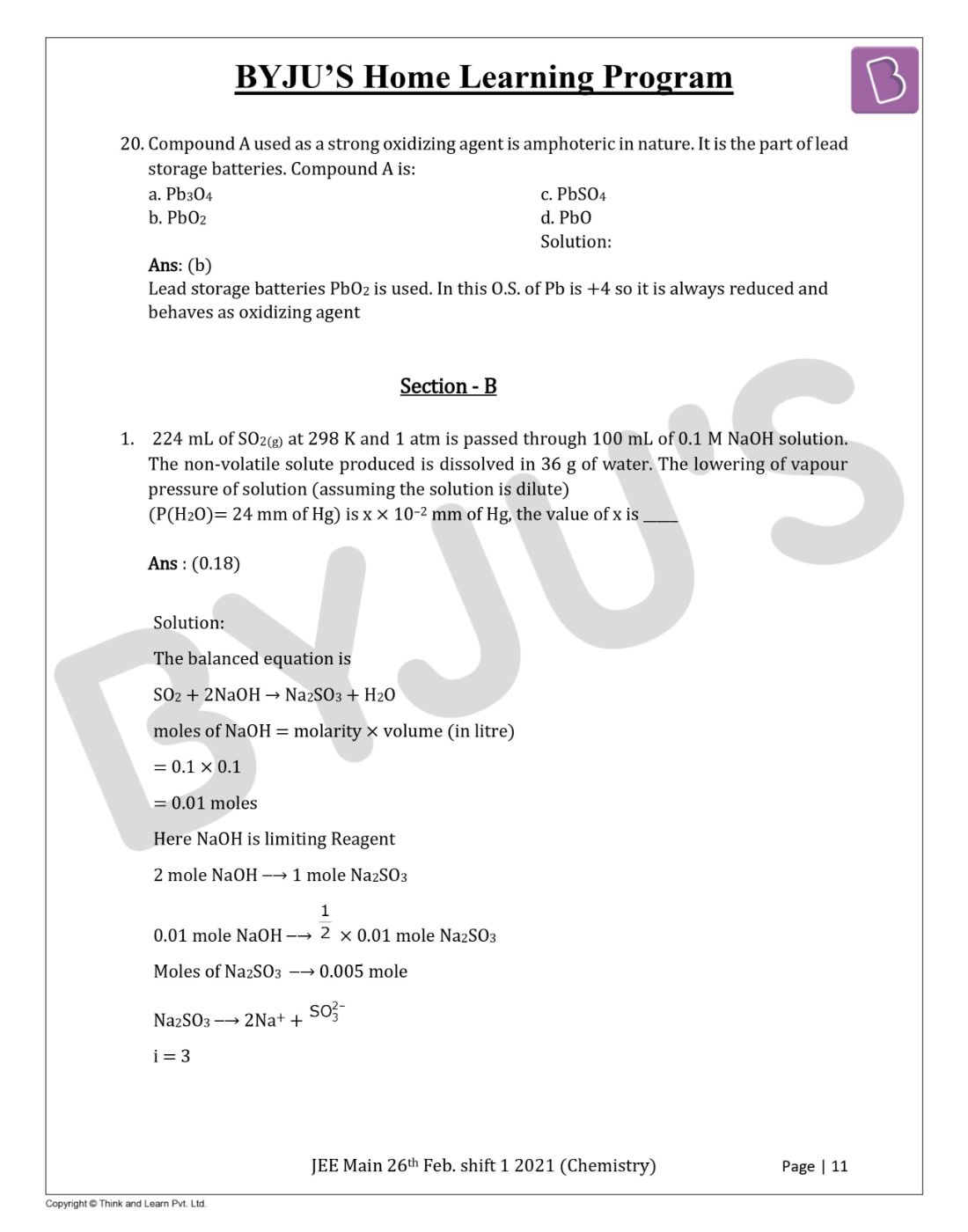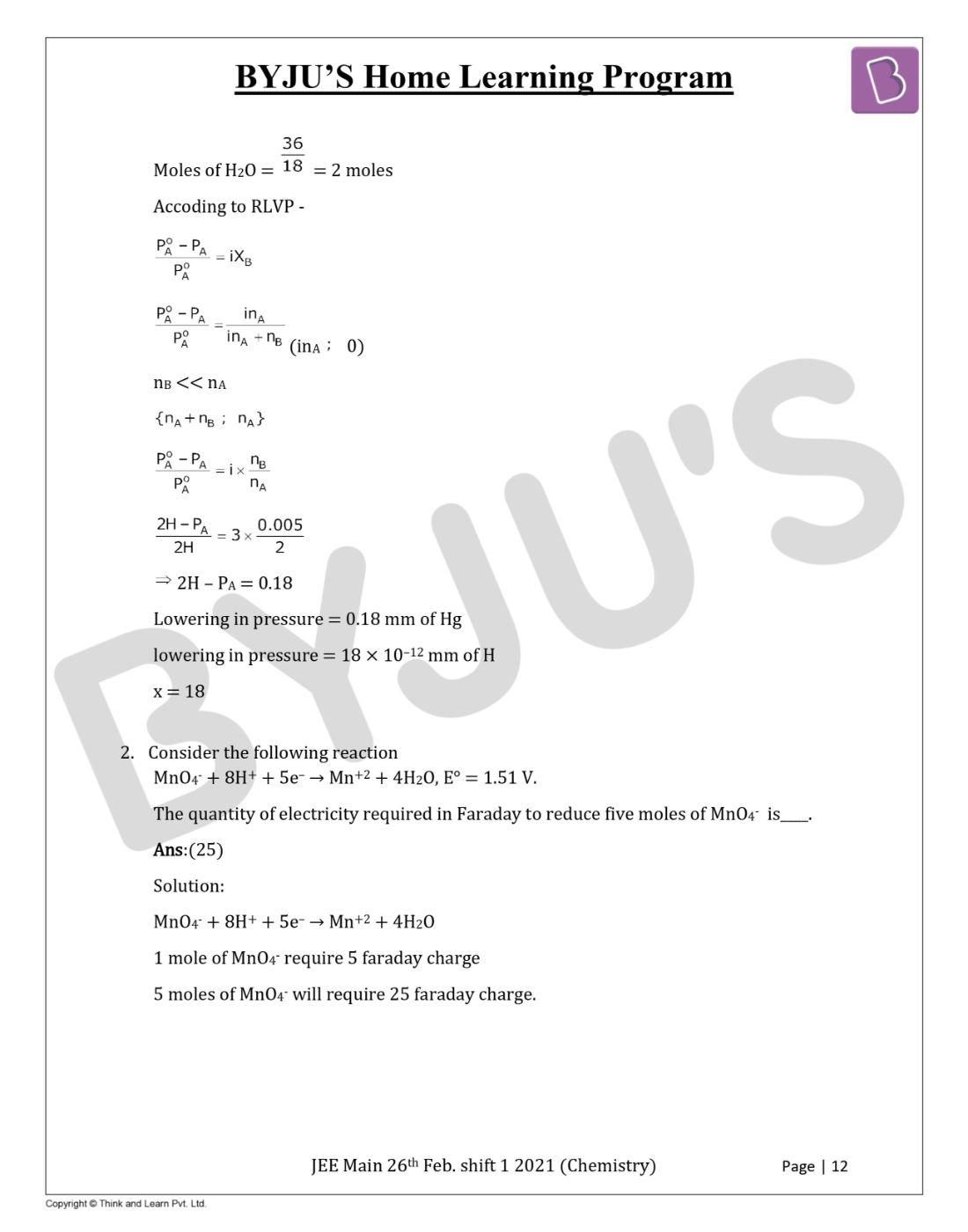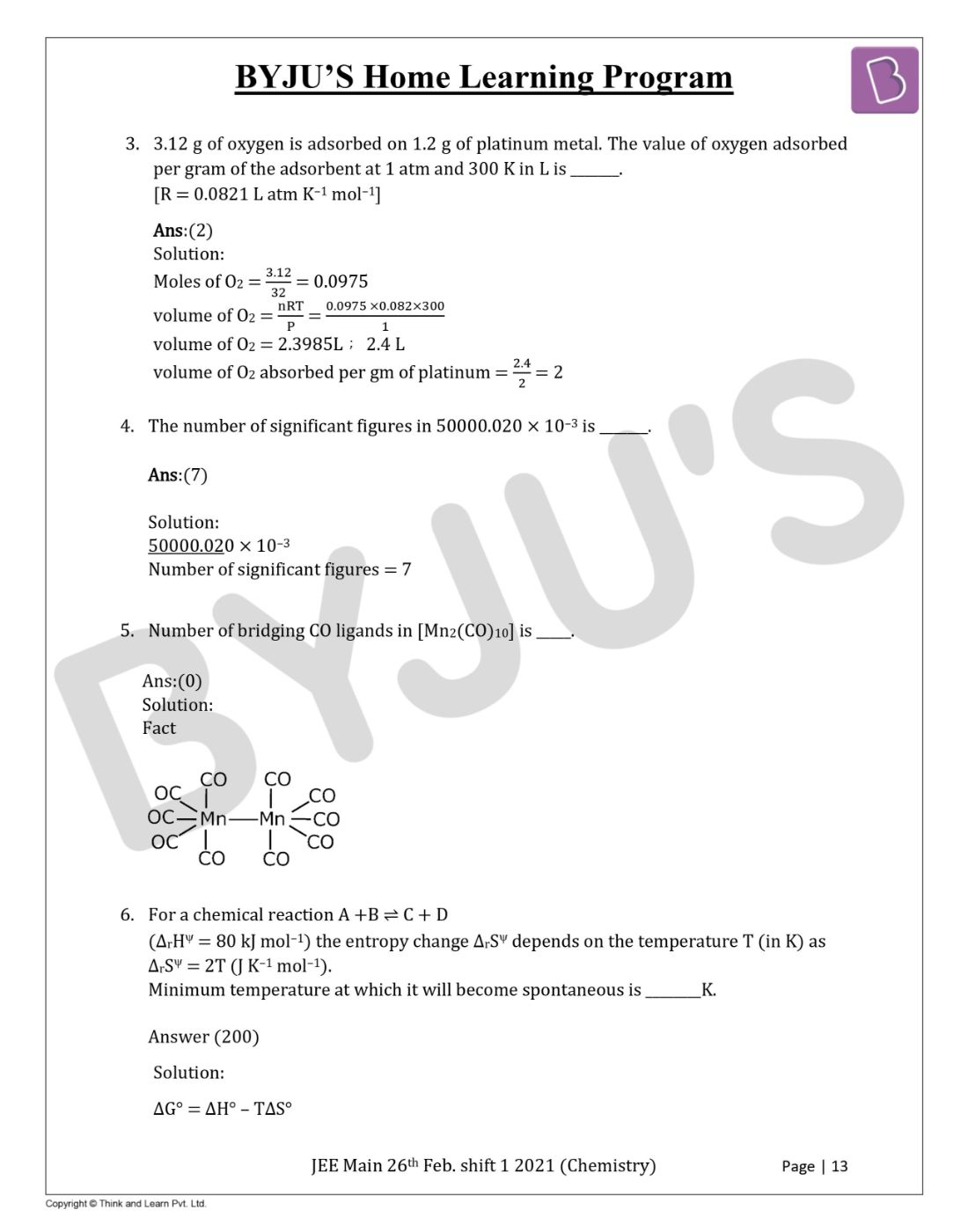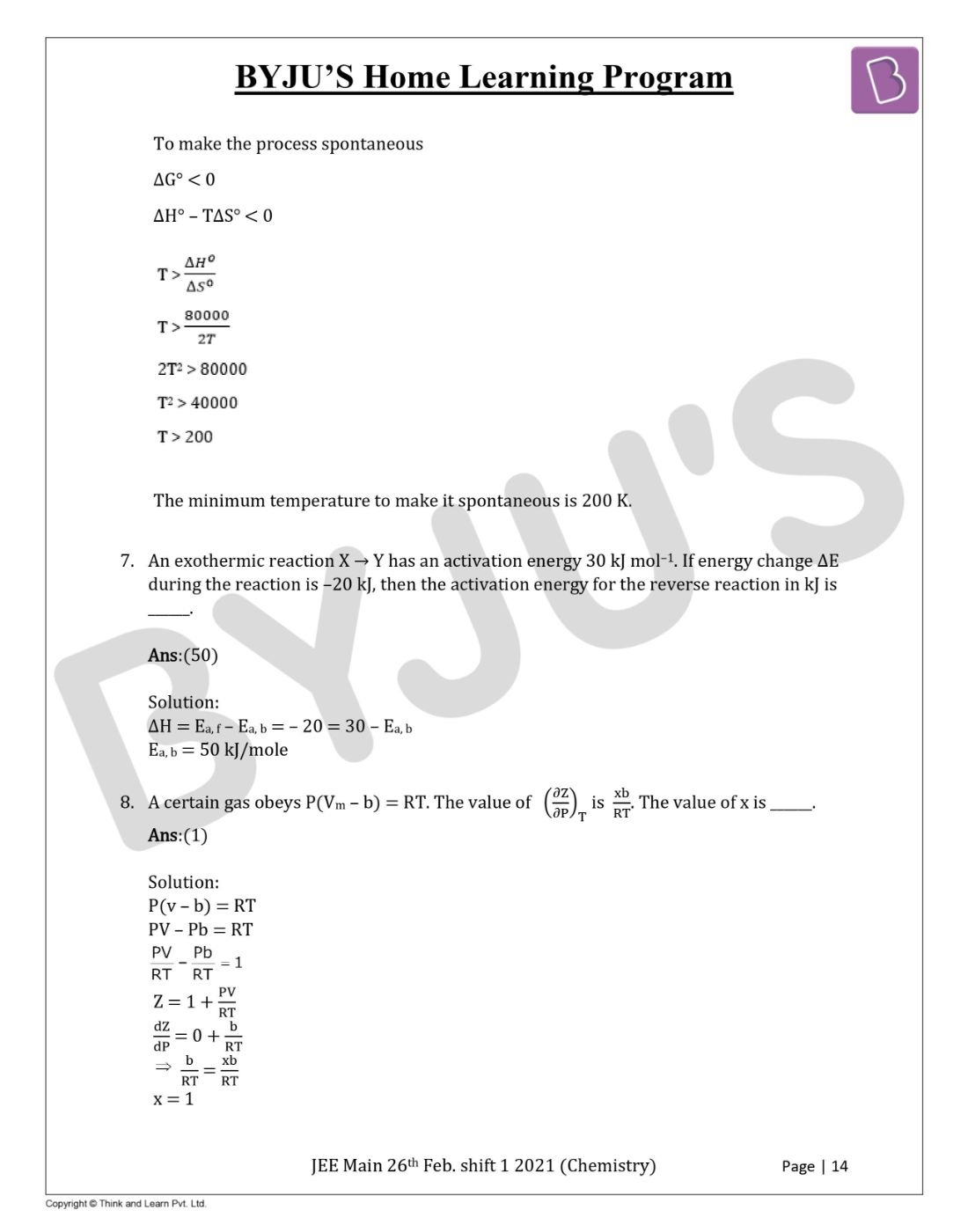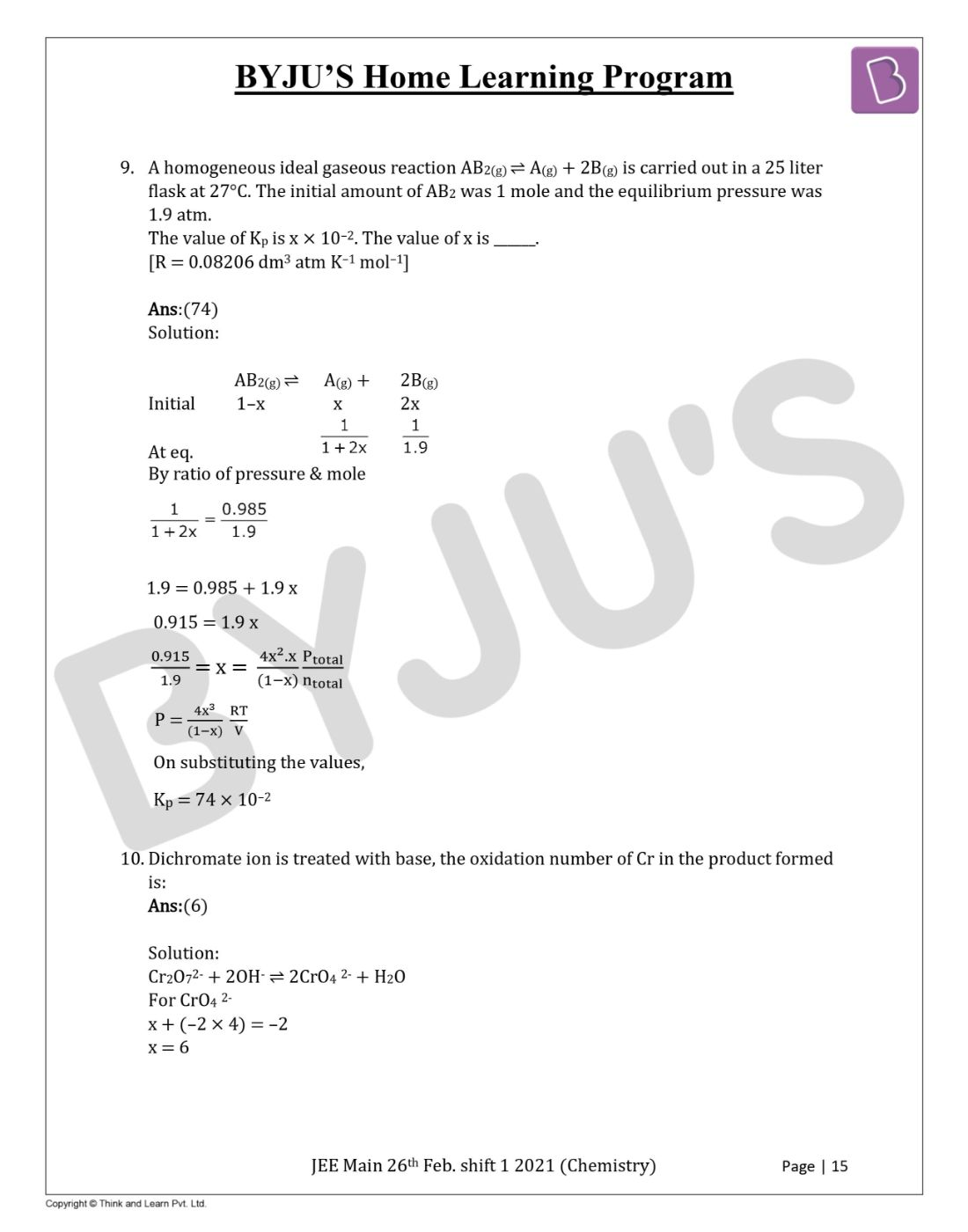Gauge your potential with Aakash BYJU'S JEE Main'23 Mock Test Gauge your potential with Aakash BYJU'S JEE Main'23 Mock Test

# JEE Main 2021 February 26 – Shift 1 Chemistry Question Paper with Solutions

JEE Main 2021 shift 1 Chemistry paper solutions are available here. You can find the Chemistry memory-based questions and these will help you to check the correct answers and analyze your performance. JEE Main 2021 question paper for Chemistry (February Shift 1) is provided here along with solutions that have been created by our subject experts. Chemistry shift 1 questions were framed from the different sections. Students can have a glance at the question paper and get a better understanding of the question types, difficulty level, marking scheme. The solutions that are provided will help candidates to find accurate answers.
JEE Main 2021 26th February Shift 1 Chemistry Question Paper

SECTION A

Question 1: B reacts with Hydroxyl amine but does not give Tollens test. Identify A and B.a. 1,1-Dichlorobutane and 2-Butanone
b. 2,2- Dichlorobutane and Butan-2-one
c. 2,2- Dichlorobutane and Butanal
d. 1,1- Dichlorobutane and Butanal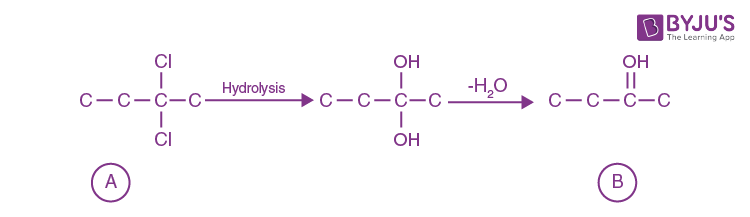Compound ‘B’ does not give Tollen’s test due to presence of kenotic group but react with hydroxyl amine.

Question 2: Match List-I with List-II.

List –I List-II

(Ore) (Element Present)

(a) Kernite (i) Tin

(b) Cassiterite (ii) Boron

(c) Calamine (iii) Fluorine

(d) Cryolite (iv) Zinc

a. (a) – (ii), (b) – (iv), (c) – (i), (d) – (iii)
b. (a) – (ii), (b) – (i), (c) – (iv), (d) – (iii)
c. (a) – (i), (b) – (iii), (c) – (iv), (d) – (ii)
d. (a) – (iii), (b) – (i), (c) – (ii), (d) – (iv)

Fact

Question 3: For the given reaction: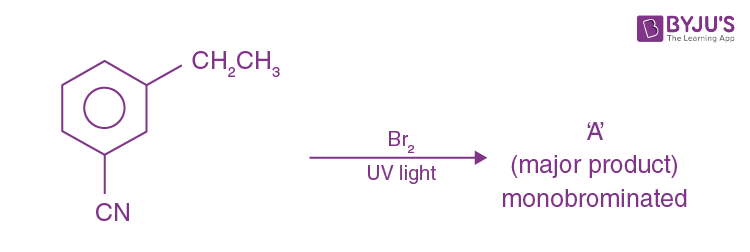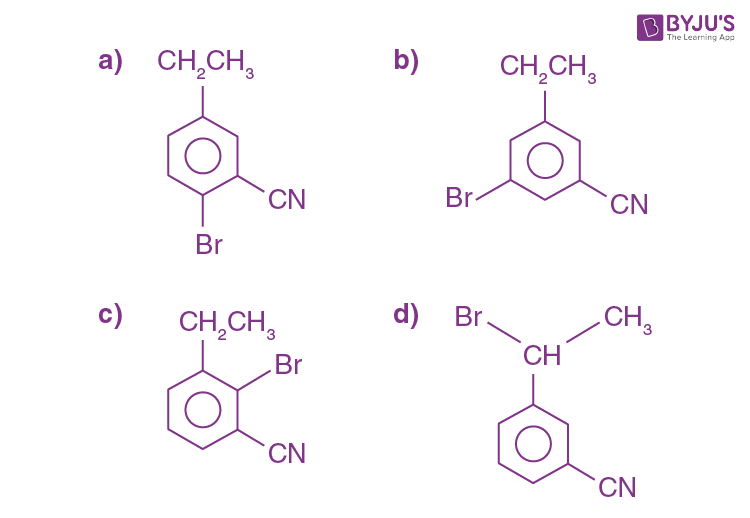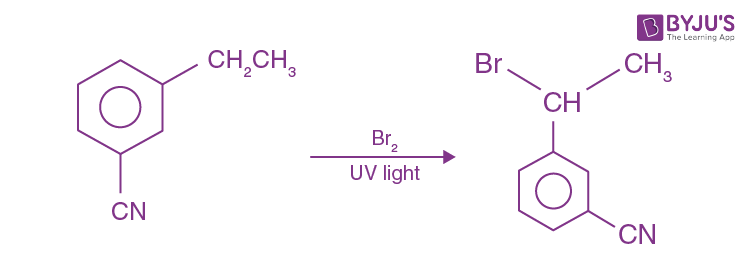It is benzylic substitution reaction.

Question 4: The orbital having two radial as well as two angular nodes is

a. 5d
b. 4f
c. 3p
d. 4d

A.N. = l

R.N = n – □ – 1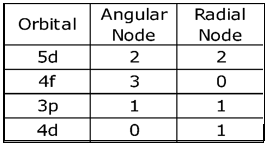Question 5: Given below are two statement:

Statement I: o-Nitrophenol is steam volatile due to intramolecular hydrogen bonding

Statement II: o-Nitrophenol has high melting point due to hydrogen bonding.

In the light of the above statements, choose the most appropriate answer from the options given below:

a. Both Statement I and Statement II are false
b. Statement I is false but Statement II is true
c. Both Statement I and Statement II are true
d. Statement I is true but Statement II is false

o-Nitrophenol is steam volatile due to intramolecular hydrogen H-bonding. but m-Nitrophenol has more melting point due to its symmetry.

Question 6: An amine on reaction with benzenesulfonyl chloride produces a compound insoluble in alkaline solution. This amine can be prepared by ammonolysis of ethyl chloride. The correct structure of amine is: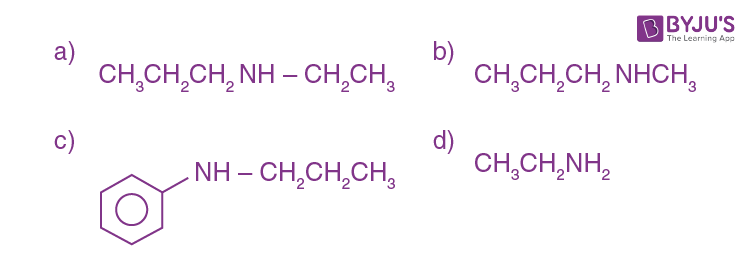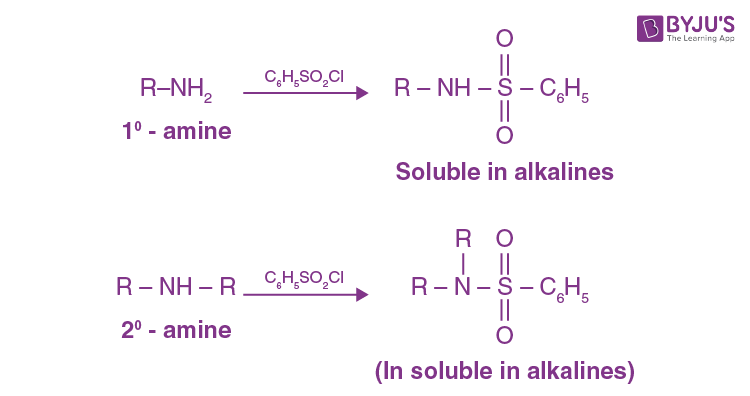According to the question the amine should be 2°- amine, in which one of the alkyl group should be ethyl, because it can be formed by ammonolysis of ethyl chloride.

Question 7: For the given reaction: What is A?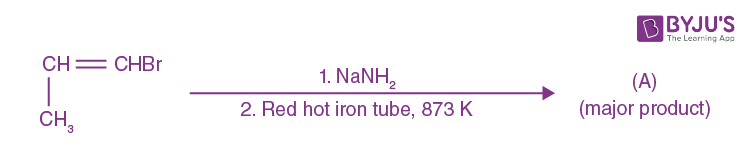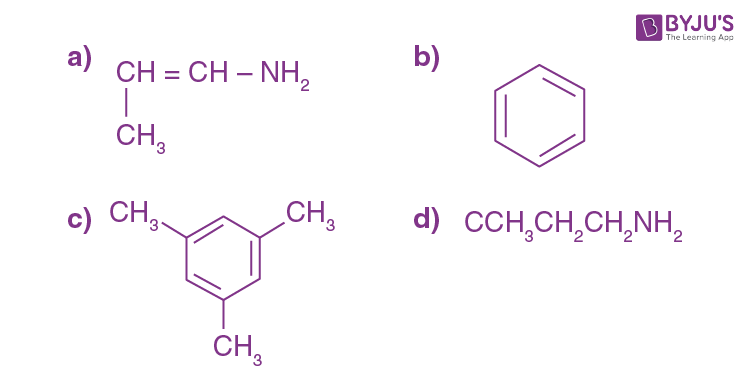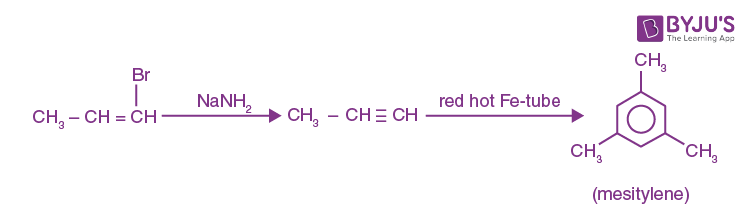Question 8: Statement about heavy water are given below

A. Heavy water is used in exchange reactions for the study of reaction mechanisms

B. Heavy water is prepared by exhaustive electrolysis of water

C. Heavy water has higher boiling point than ordinary water

D. Viscosity of H2O is greater than D2O

a. A and B only
b. A and D only
c. A, B and C only
d. A and C only

Fact

Question 9: Which of the following is ‘a’ FALSE statement?

a. Carius tube used in the estimation of sulphur in an organic compound (i) and (iv) are isotopes
b. Kjeldahl’s method is used for the estimation of nitrogen in an organic compound (ii) and (iii) are isotopes
c. Phosphoric acid produced on oxidation of phosphorus present in an organic compound is precipitated as Mg2P2O7 by adding magnesia mixture
d. Carius method is used for the estimation of nitrogen in an organic compound

Fact

Question 10: Given below are two statements:

Statement I: A mixture of chloroform and aniline can be separated by simple distillation

Statement II: When separating aniline from a mixture of aniline and water by steam distillation aniline boils below its boiling point

In the light of the above statements, choose the most appropriate answer from the options given below:

a. Statement I is true, statement II is false
b. Both Statement I and Statement II are true
c. Both Statement I and Statement II are false
d. d. Statement I is false, Statement II is true

A suitable method for separating a mixture of aniline and chloroform would be steam distillation. Steam distillation is the process used to separate aromatic compound from a mixture because of their temperature sensitivity. Therefore, steam distillation is an ideal method for their separation.

Question 11: Which of the following vitamin is helpful in delaying the blood clotting?

a. Vitamin B
b. Vitamin C
c. Vitamin K
d. Vitamin E

Vitamin K is used by the body to help blood clot.

Question 12: The presence of ozone in troposphere:

a. Generates photochemical smog
b. Protects us from the UV radiation
c. Protects us from the X-ray radiation
d. Protects us from greenhouse effect

The presence of ozone in troposphere protect earth from ultraviolet ray.

Question 13: On treating a compound with warm dil. H2SO4, gas X is evolved which turns K2Cr2O7 paper acidified with dil. H2SO4 to a green compound Y. X and Y respectively are:

a. X = SO2, Y = Cr2 (SO4)3
b. X = SO2, Y = Cr2O3
c. X = SO3, Y = Cr2O3
d. X = SO3, Y = Cr2 (SO4)3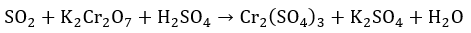Question 14: Find A, B and C in the following reaction:

NH3 + A + CO2 → (NH4)2CO3

(NH4)2CO3 + H2O + B → NH4HCO3

NH4HCO3 + NaCl → NH4Cl + C

a. A – H2O; B – CO2; C – NaHCO3
b. A – H2O; B – O2; C – Na2CO3
c. A – O2; B – CO2; C – Na2CO3
d. A – H2O; B – O2; C – NaHCO3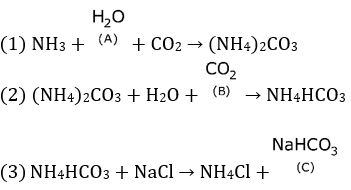Question 15: Given below are two statements: one is labelled as Assertion A and the other is labelled as Reason R.

Assertion A: Dipole-dipole interactions are the only non-covalent interactions, resulting in hydrogen bond formation

Reason R: Fluorine is the most electronegative element and hydrogen bonds in HF are symmetrical

In the light of the above statements, choose the most appropriate answer from the options given below:

a. A is false but R is true
b. Both A and R are true and R is the correct explanation of A
c. A is true but R is false
d. Both A and R are true and R is not the correct explanation of A

Fact

Question 16: Match List-I with List-II.

List –I List-II

Electronic configuration of elements ΔiH in kJ mol–1

(a) 1s22s2 (i) 801

(b) 1s22s22p4 (ii) 899

(c) 1s22s22p3 (iii) 1314

(d) 1s22s22p1 (iv) 1402

a. (a) – (ii), (b) – (iii), (c) – (iv), (d) – (i)
b. (a) – (iv), (b) – (i), (c) – (ii), (d) – (iii)
c. (a) – (i), (b) – (iv), (c) – (iii), (d) – (ii)
d. (a) – (i), (b) – (iii), (c) – (iv), (d) – (ii)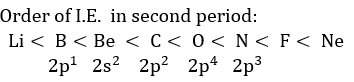Question 17: Which one of the following lanthanoids does not form MO2?

[M is lanthanoid metal]

a. Nd
b. Yb
c. Dy
d. Pr

Fact

Question 18: Identify the major products A and B respectively in the following reaction of phenol: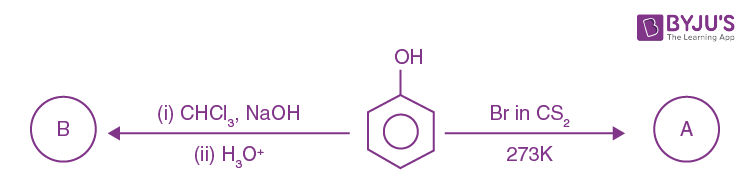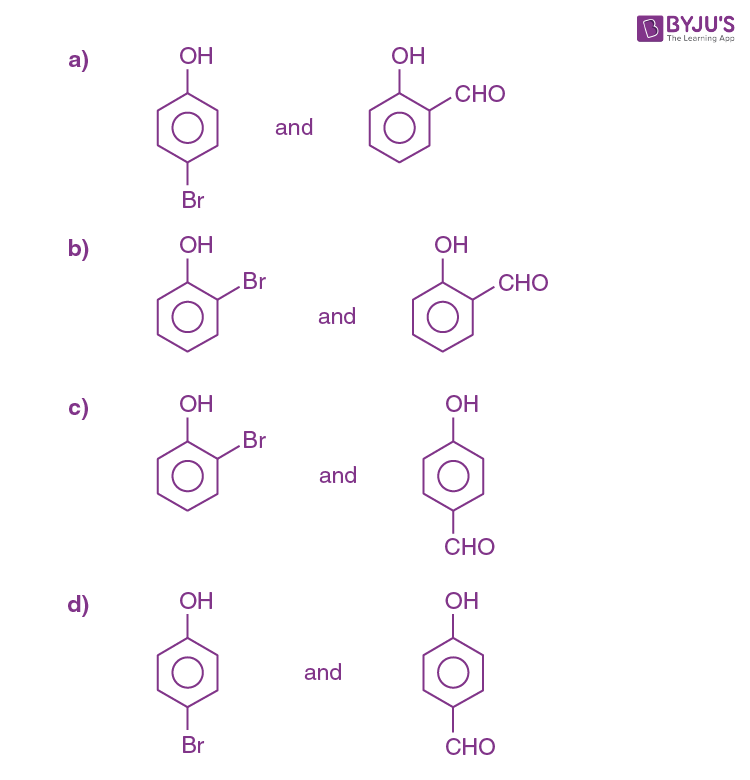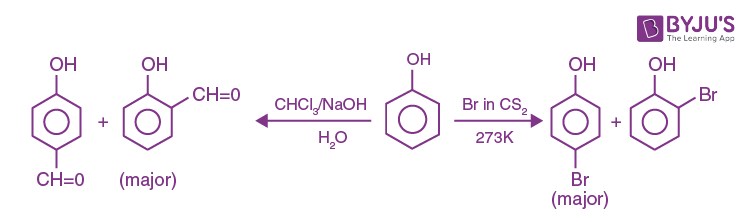Question 19: The structure of Neoprene is: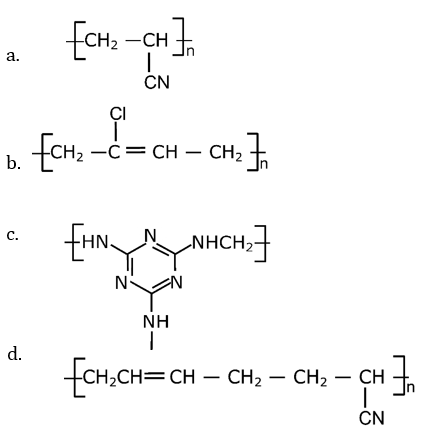Question 20:Compound A used as a strong oxidizing agent is amphoteric in nature. It is the part of lead storage batteries. Compound A is:

a. Pb3O4
b. PbO2
c. PbSO4
d. PbO

Lead storage batteries PbO2 is used. In this O.S. of Pb is +4 so it is always reduced and behaves as oxidizing agent.

Section B

Question 1: 224 mL of SO2(g) at 298 K and 1 atm is passed through 100 mL of 0.1 M NaOH solution. The non-volatile solute produced is dissolved in 36 g of water. The lowering of vapour pressure of solution (assuming the solution is dilute) (P(H2O)= 24 mm of Hg) is 10–2 x mm of Hg, the value of x is _____

The balanced equation is

SO2 + 2NaOH → Na2SO3 + H2O

moles of NaOH = molarity × volume (in litre)

= 0.1 × 0.1

= 0.01 moles

Here NaOH is limiting Reagent

2 mole NaOH → 1 mole Na2SO3

0.01 mole NaOH → (1/2) × 0.01 mole Na2SO3

Moles of Na2SO3 ⎯→ 0.005 mole

Na2SO3 → 2Na+ + SO2-3

i = 3

Moles of H2O = (36/18) = 2 moles

According to RLVP –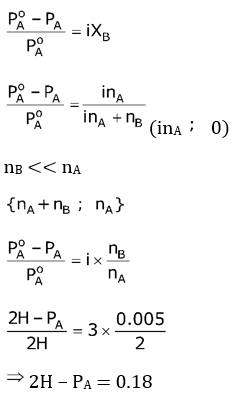Lowering in pressure = 0.18 mm of Hg

lowering in pressure = 18 × 10–12 mm of H

x = 18

Question 2: Consider the following reaction

MnO4 + 8H+ + 5e → Mn+2 + 4H2O, Eo = 1.51 V.

The quantity of electricity required in Faraday to reduce five moles of MnO4 is____.

MnO4 + 8H+ + 5e → Mn+2 + 4H2O

1 mole of MnO4 require 5 faraday charge

5 moles of MnO4 will require 25 faraday charge

Question 3: 3.12 g of oxygen is adsorbed on 1.2 g of platinum metal. The value of oxygen adsorbed per gram of the adsorbent at 1 atm and 300 K in L is _______.

[R = 0.0821 L atm K–1 mol–1]

Moles of O2 = 3.12/32 = 0.0975

volume of O2 = nRT/P = (0.0975 ×0.082×300)/1

volume of O2 = 2.3985L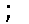2.4 L

volume of O2 absorbed per gm of platinum = 2.4/2 = 2

Question 4: The number of significant figures in 50000.020 × 10–3 is _______.

50000.020 × 10–3

Number of significant figures = 7

Question 5: Number of bridging CO ligands in [Mn2(CO)10] is _____.

Fact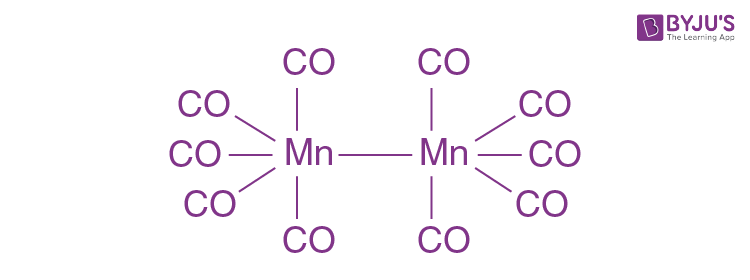Question 6: For a chemical reaction A +B ⇌ C + D rHψ = 80 kJ mol–1) the entropy change ΔrSψ depends on the temperature T (in K) as ΔrSψ = 2T (J K–1 mol–1). Minimum temperature at which it will become spontaneous is ________K.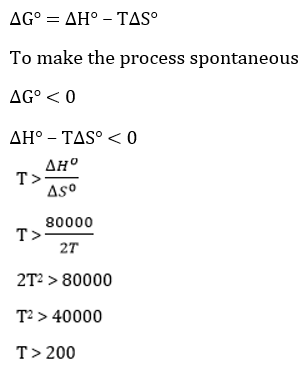The minimum temperature to make it spontaneous is 200 K.

Question 7: An exothermic reaction X → Y has an activation energy 30 kJ mol–1. If energy change ΔE during the reaction is –20 kJ, then the activation energy for the reverse reaction in kJ is ______.

ΔH = Ea, f – Ea, b = – 20 = 30 – Ea, b

Ea, b = 50 kJ/mole

Question 8: A certain gas obeys P(Vm – b) = RT. The value of (∂Z/∂P)T is xb/RT. The value of x is ______.

P(v – b) = RT

PV – Pb = RT

Z = 1 + PV/RT

dZ/dP = 0 + b/RT

b/RT = xb/RT

x = 1

Question 9: A homogeneous ideal gaseous reaction AB2(g) ⇌ A(g) + 2B(g) is carried out in a 25 liter flask at 27oC. The initial amount of AB2 was 1 mole and the equilibrium pressure was 1.9 atm. The value of Kp is x × 10–2. The value of x is ______. [R = 0.08206 dm3 atm K–1 mol–1]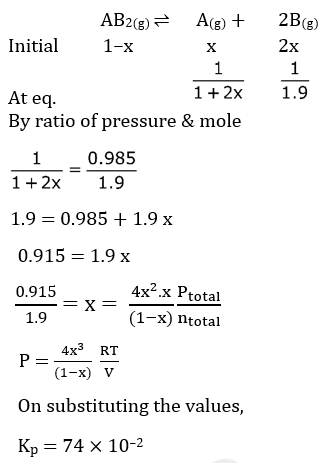Question 10: Dichromate ion is treated with base, the oxidation number of Cr in the product formed is:

Cr2O72- + 2OH ⇌ 2CrO42- + H2O

For CrO42-

x + (–2 × 4) = –2

x = 6